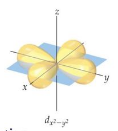# Problem: How would the dx2- y2 orbital in the n=5 shell compare to the dx2-y2 orbital in the n=3 subshell?a) The contour of the orbital would extend further out along the x and y axes.b) The value of ℓ  would increase by 2.c) The radial probability function would include two more nodes.d) The orientation of the orbital would be rotated 45° along the xy plane.e) The mℓ value would be the same.

###### FREE Expert Solution

We are asked to determine how would the dx2- y2 orbital in the n=5 shell compare to the dx2-y2 orbital in the n=3 subshell

Below is a general representation of the said orbital:94% (272 ratings)###### Problem Details

How would the dx2- y2 orbital in the n=5 shell compare to the dx2-y2 orbital in the n=3 subshell?

a) The contour of the orbital would extend further out along the x and y axes.

b) The value of ℓ  would increase by 2.

c) The radial probability function would include two more nodes.

d) The orientation of the orbital would be rotated 45° along the xy plane.

e) The mℓ value would be the same.